# 【NLP】Conditional Language Modeling with Attention

Review: Conditional LMs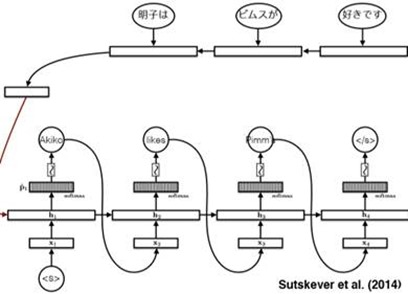Note that, in the Encoder part, we reverse the input to the ‘RNN’ and it performs well.

And we use the Decoder network(also a RNN), and use the ‘beam search’ algorithm to generate the target statement word by word.

The above network is a translation model.But it still needs to optimizer.

A very essential part of the model is the [Attention mechanism].

Conditional LMs with Attention

In last blog, we compress a lot of information in a finite-sized vector and use it as the condition. That is to say, in the ‘Decoder’, for each input we use this vector as the condition to predict the next word.

But is it really correct?

An obvious thing is that a finite-sized vector cannot contain all the information since the input sentence could have a very one length. And gradients have a long way to travl so even LSTMs could forget!

In Translation Question, we can solve the problem by this:

Represent a source sentence as a matrix whose size can be changeable.

Then Generate a target sentence from the matrix. (As the condition and the condition is transformed form that matrix)

So how does this do?

The very simpal way to fulfill that is [With Concatenation].

We have already known that the words can be represented by ‘embedding’ such as Word2Vec. And all the embeddings have the same size. For a sentence composed by n words, we can just put each word’s embedding together. So the matrix size is |vocabulary size|*n, which n is the length of sentence. That’s a really easy solution but it is useful. E.g.Another solution proposed by Gehring et al. (2016,FAIR) is [With Convolutional Nets].

It is to say, we use all embedding of the word from the sentence to form the concatenation matrix (just like the above method), and then we use a CNN to handle this matrix using some filters. And final we also generate a new matrix to represent the information. And in my opinion, this is a bit like extracting advanced features from image processing. E.g.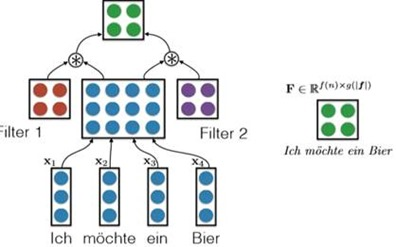The most important method is [using the Bidirectional RNNs].

For one side, we use a RNN to handle the embedding, and we get n hidden layers which n is the length of the word.

For another side, we use another RNN to handle the embedding, but we reverse the input and finally we also get n hidden layers.

We put the 2n hidden layers together to generate the conditional matrix. E.g.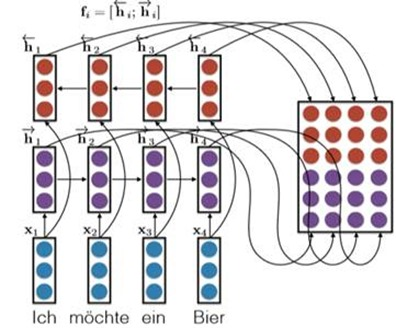There are some other ways needed to be founded.

So next to the important part: how to use the ‘Attention model’ and use the attention to generate the condition vector form the condition matrix F.

Firstly, considering the decoder RNN: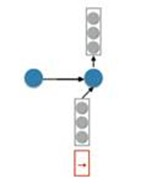We have a ‘start hidden layer’ and then generate the next hidden layer using the input x and we still need a conditional vector.

Suppose we also had an attention vector a. We can generate the condition vector by doing this:

c = Fa. Where F is the matrix and a is the attention vector. This can be understood as weighting the conditional matrix so that we can pay more attention to the contents of a certain sentence.

E.g.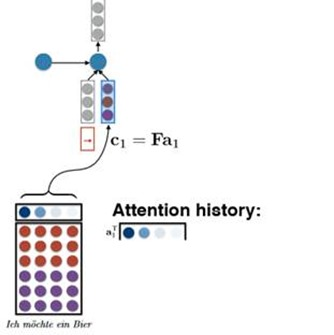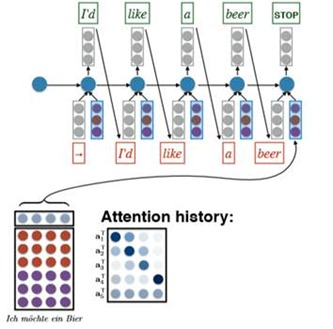So How to generate the Attention Vector?

That is, how do we compute a.

We can do by the following method:

For the time t, we know the hidden layer Ht-1, and we do linear transformation to it to generate a vector r. ( r = VHt-1) V is the learned parameter. Then we take dot product with every column in the source matrix to compute the attention energy a. ( a = F.T*r). So we generate the attention vector a by using a softmax to Exponentiate and normalize it to 1.

That is a simpliﬁed version of Bahdanau et al.’s solution. Summary of it:

Another complex way to generate the attention vector is to use the [Nonlinear Attention-Energy Model].

Getting the r above, ( r = VHt-1) we generate a by: a = v.T * tanh(WF + r). Where v W and V is the learned parameter. How useful of the r is not to verify.

Summary

We put it all together and this is called the conditional LM with attention.

Attention in machine translation.

Add attention to seq2seq model translation: +11 BLEU.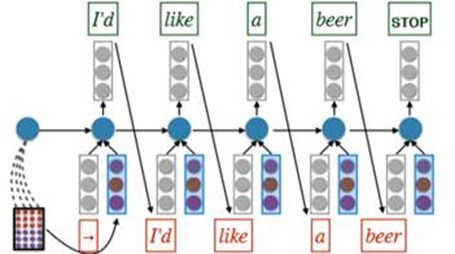An improvement in computing: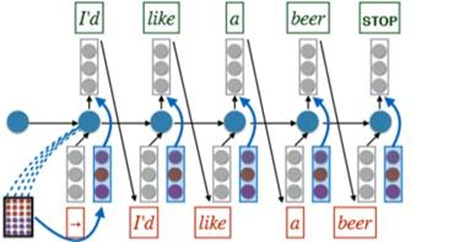Note the difference form the above model. But whether it is useful is not sure.Comprehension

Cho’s question: does a translator read and memorize the input sentence/document and then generate the output?

• Compressing the entire input sentence into a vector basically says “memorize the sentence”

• Common sense experience says translators refer back and forth to the input. (also backed up by eyetracking studies)

Image caption generation with attention: brief introduction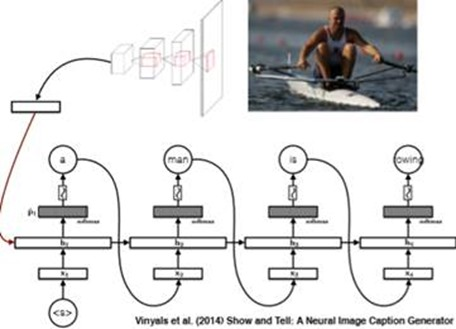The main idea is that: we encode the picture to a matrix F and use it generate some attention and finally use the attention to generate the caption.

Generate matrix F: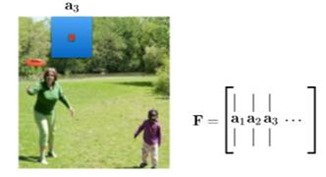Attention “weights” (a) are computed using exactly the same technique as discussed above.

Other techinques: Stochastic hard attention(sampling matrix F idea and not like the weighting matrix F idea). Learning Hard Attention. To be honesty, I don't know much about this.

posted @ 2018-08-02 21:30  pigcv  阅读(456)  评论(0编辑  收藏  举报